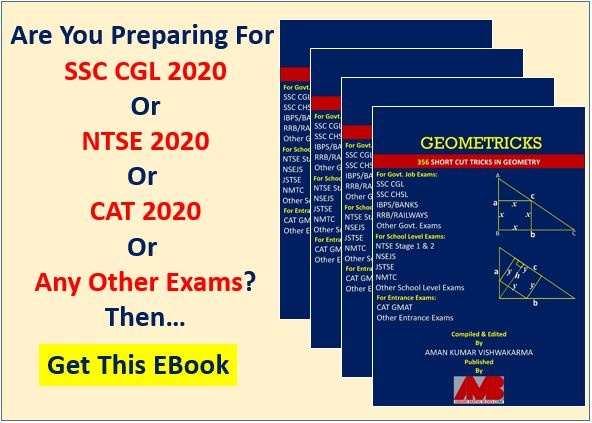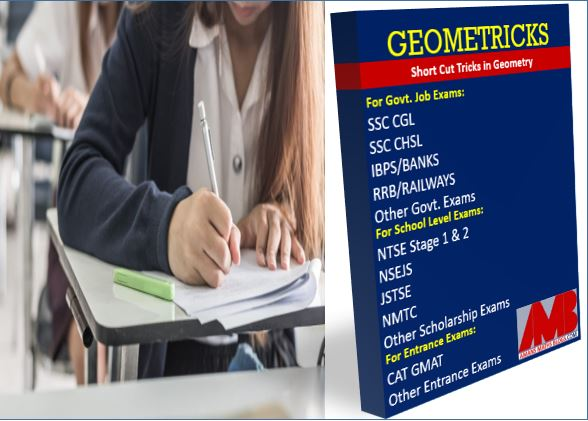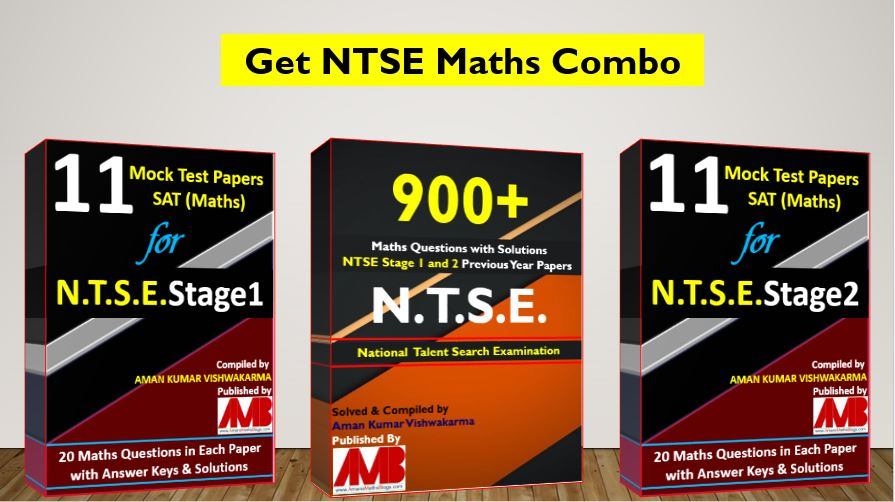Monday, September 27, 2021
Home > Latest Announcement > All Short Tricks In Geometry | Geometricks EBook

# All Short Tricks In Geometry | Geometricks EBook# All Short Tricks In Geometry | Geometricks EBook

Hi students, welcome to AmansMathsBlogs (AMB). This is an EBook of Geometry known as GEOMETRICKS, it contains about 356 Short tricks of Geometry concepts. Get All Short Tricks in Geometry Formulas in a PDF format.

Are you preparing for competitive exams in 2020 like SSC CGL, SSC CHSL and other SSC exams, IBPS Bank Exams, RRB Railways Exams or any other government jobs exams or school level exams like NTSE Stage 1 and 2, NSEJS, JSTSE, NMTC or any CBSE level exams or CAT, GMAT or any other entrance exams?In all of these exams, a math paper contains complicated and tricky geometry questions which require more concept, tips and trick in geometry.

So I write an ebook for geometry that contains all short tricks in geometry and named this ebook as GEOMETRICKS. In this ebook, there are total of 356 geometry short cut tricks included. The 356 short tricks of geometry includes the following modules:

To get NMTC 2004 to 2018 Questions with Solutions, Click Image Below

Module 1: BASIC GEOMETRY

In this module, the tips and tricks of basic geometry like number of regions, number of intersection points, number of parallelograms and other basic definitions are included.

Module 2: POLYGONS

In this module, the tips and tricks of polygons like area, circumradius and number of triangles common side with polygons and other properties of polygons are included.

Module 3: TRIANGLES

In this module, the tips and tricks of triangles like number of acute and obtuse angles, isosceles within isosceles, Cevian formulas, Stewart theorem, Routh theorems and other tips and tricks of triangles are included.

In this module, the tips and tricks of quadrilaterals like triangle within parallelogram, square within square, mid-point formula and other tips and tricks of quadrilaterals are included.

Module 4: CIRCLE

In this module, the tips and tricks of circles like length of common chords, results of two perpendicular chords, Ptolemy theorem for any quadrilateral, circles within circle  and other tips and tricks of circles theorems are included.

Module 5: MENSURATION

In this module, the tips and tricks of mensuration like perimeter, area of 2D figures and surface area and volume of 3D figures, prism, pyramid, sphere within cone, cylinder  and other tips and tricks of mensuration formulas are included.

Module 5: MASS POINT GEOMETRY

In this module, the tips and tricks of cevian based question solving techniques by mass point geometry (MPG) method and other tips and tricks of MPG are included.

After combining all the concepts, tips and tricks of these five modules, there are total of 356 concepts and tips and tricks are included in this GEOMETRICKS EBook PDF.

This EBook is the file size of 6.87 MB, total number of pages is 219, total number of words is 24607.

Now, in GEOMETRICKS Ebook, there are more than 300 (including TOP 120 Geometry Concepts Tips Tricks For Competitive Exams,) concepts tips and tricks are written.To get this GEOMETRICKS Ebook, click RED button below.

## NTSE Maths Combo Pack EBook

Get 900+ NTSE Maths Previous Year Question Bank with Solutions and 11 Mock Test Papers EBook for NTSE exams. For more details, Click To NTSE Stage 1 Mock Test SAT Maths and NTSE Stage 2 Mock Test SAT Maths. To get this combo ebook, click below RED button.Get Online Maths Tuition by AMBiPi for Class 8 to 12